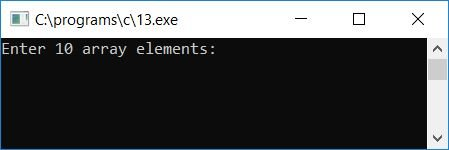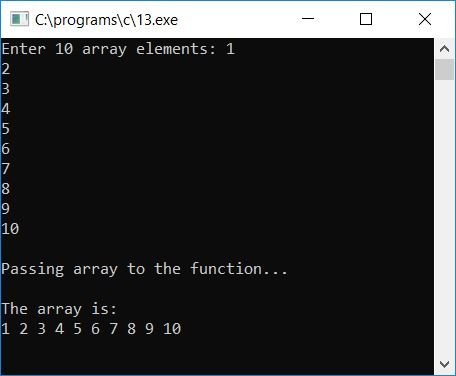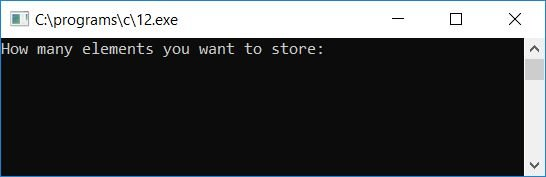# Passing an array to a function in C

In this article, we will learn how to pass an individual array element or whole array to a function in C. First, we have created a program that passes an entire array to a function. And then I created a program that passes individual array elements to a function.

## Passing the entire array to function

Let's learn, with the program given below, how to pass a whole array to any function in C. The passed array can be used in functions just like it can be used in the main function.

```#include<stdio.h>
#include<conio.h>
void func(int arr[]);
int main()
{
int arr, i;
printf("Enter 10 array elements: ");
for(i=0; i<10; i++)
scanf("%d", &arr[i]);
printf("\nPassing array to the function...\n");
func(arr);
getch();
return 0;
}
void func(int arr[])
{
int i;
printf("\nThe array is:\n");
for(i=0; i<10; i++)
printf("%d ", arr[i]);
}```

As you can see from the above program, the whole array (everything inside the array) gets passed into a function named func(), which performs the operation.

The above program was written inside the Code::Blocks IDE; therefore, after a successful build and run, here is the sample output:Now provide any 10 array elements (numbers) and then press the ENTER key. After this, you will get a message saying "Passing array to the function," and then the complete array gets passed to the function named func(). Inside that function, all the 10 entered array elements get printed back on the output screen; here is the second screenshot of the sample run:#### Program Explained

• Receive any 10 numbers as 10 array elements and store them inside the array, saying arr[], one by one.
• That is, the first number is stored in arr, the second number in arr, the third number in arr,... and the tenth number in arr.
• Now pass the array to the function named func().
• To pass an array to a function, just write the name of the array as an argument of the function, that is, func(arr), where func() is the name of the function and arr is the name of the array.
• Never forget to declare the function before the main() function or before calling the function.
• With the func(arr); statement, the array arr gets passed to the function definition portion of the program.
• Inside the function definition, we have printed back all 10 elements of the array arr on the output screen.

## Passing the individual array elements to a function in C

Now let's create another program that passes individual array elements to a function one by one.

```#include<stdio.h>
#include<conio.h>
void check(int num);
int main()
{
int arr, i;
printf("Enter 10 Array elements:\n");
for(i=0; i<10; i++)
scanf("%d", &arr[i]);
printf("\nChecking each elements for Even/Odd..\n");
for(i=0; i<10; i++)
check(arr[i]);
getch();
return 0;
}
void check(int num)
{
if(num%2==0)
printf("%d is Even\n", num);
else
printf("%d is Odd\n",num);
}```

As you can see here, all 10 elements of the array get passed to the function check(), which checks whether the element is an even number or an odd number. Here is its sample run:Now enter all 10 numbers (array elements) and then press ENTER. Here is the sample output:#### Program Explained

• Receive any 10 array elements.
• Assume the user has supplied array elements such as 1, 2, 3,..., 10.
• Therefore, the first number, which is 1, gets stored at arr, the second number, which is 2, gets stored at arr, the third number, which is 3, gets stored at arr,... and the tenth element, which is 10, gets stored at arr.
• Now we have printed a message: checking each element to see if it is even or odd.
• Following this message, we created a for loop that runs from 0 to 9 in order to check all 10 elements of the array that are present at index numbers 0, 1, 2,..., and 9.
• On the first run of the for loop, check(arr[i]) or check(arr) or check(1) is passed to the function check(). That is, the number 1 (the first number or number present at the 0th index of the array) gets passed to the function named check().
• Inside the function, we have used an if-else statement to check for even and odd.
• That is, if num%2 (the num variable holds the number passed through the function that is 1 this time) or 1%2 is equal to 0 or not.
• If it is, then print the number as even; otherwise, print the number as odd.
• After printing "even" or "odd," the program goes back to the main() function, where the for loop variable i is increased by 1, and if 1 is less than 10, the program goes back to the for loop a second time.
• The function check(arr[i]), check(arr, or check(2) is called again. That is the number that gets passed to the function check().
• Inside the function, the num variable is set to 2, and it is checked to see if 2 can be divided by 2 without leaving a remainder.
• If it is, then print the number as even; otherwise, print the number as odd.
• Do the same thing until the for loop's condition evaluates to false, that is, until the value of i (the loop variable) becomes 10 and the condition i<10 or 10<10 evaluates to false, and program flow exits the loop.
• Therefore, we have passed all the given 10 array elements one by one to the function to check and print as even or odd.

Here is the modified version of the above program. The user is allowed to define the size of the array before entering elements for it:

```#include<stdio.h>
#include<conio.h>
void checkEven(int e);
void checkOdd(int o);
static int n1=0, n2=0;
int main()
{
int arr, i, size;
printf("How many elements you want to store: ");
scanf("%d", &size);
printf("Enter all %d Array elements:\n", size);
for(i=0; i<size; i++)
scanf("%d", &arr[i]);
printf("\nChecking each elements for Even/Odd..\n");
printf("\nList of all Even numbers: ");
for(i=0; i<size; i++)
checkEven(arr[i]);
printf("\nList of all Odd numbers: ");
for(i=0; i<size; i++)
checkOdd(arr[i]);
getch();
return 0;
}
void checkEven(int e)
{
if(e%2==0)
{
if(n1==0)
printf("%d", e);
else
printf(", %d", e);
n1++;
}
}
void checkOdd(int o)
{
if(o%2 != 0)
{
if(n2==0)
printf("%d", o);
else
printf(", %d", o);
n2++;
}
}```

This program is designed to ask the user at run-time how many elements he/she wants to store, say 10, and then prompt the user to enter all elements (say 10). Finally, the program will list out all the even and odd numbers. Let's take a look at its output with the sample run given below:Now enter 10 as the size of the array, then provide all the 10 elements, and then press ENTER to see all the even and odd numbers from those entered 10 numbers:Note: A variable of the static type holds or remembers its previous value throughout the program.

C Quiz

« Previous Program Next Program »